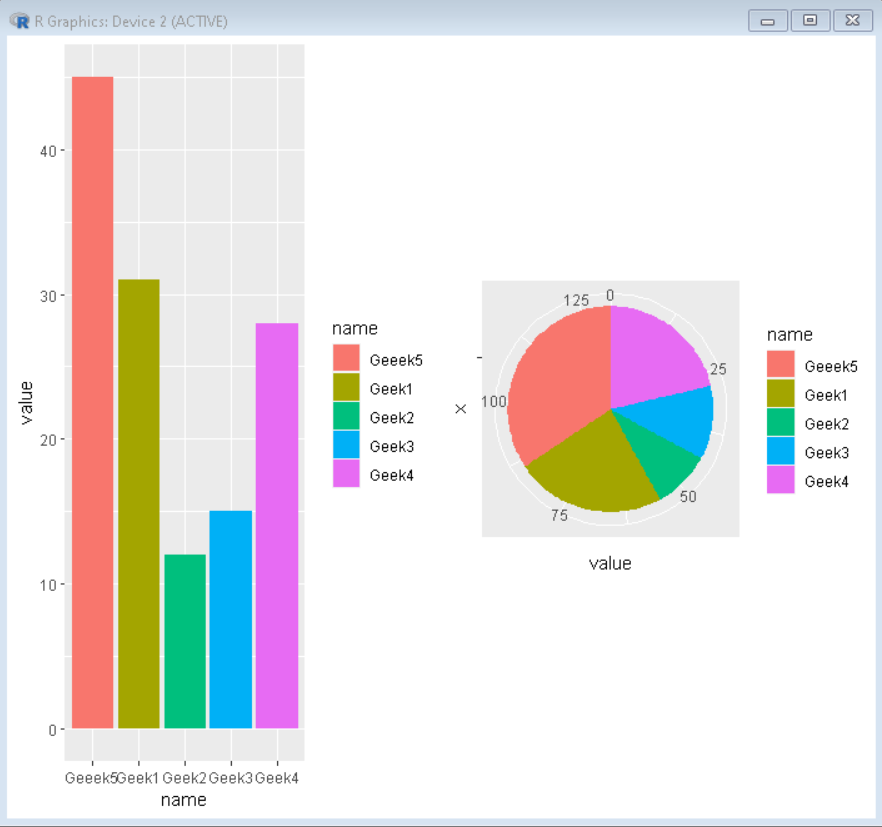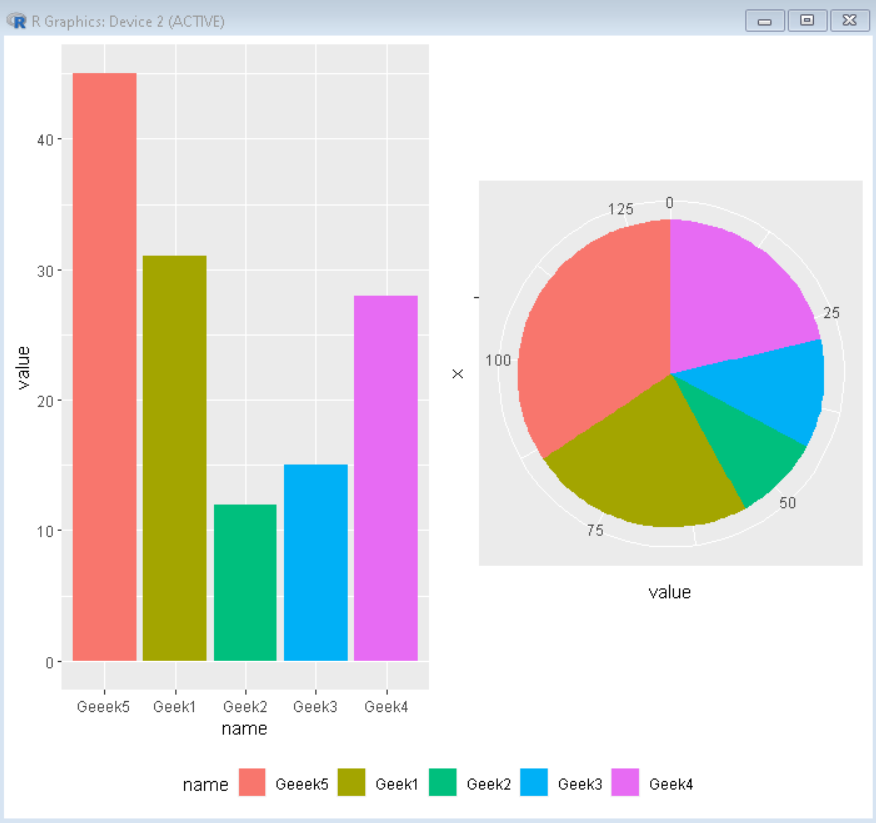# Shared legend for a cowplot grid in R

• Last Updated : 24 Oct, 2021

In this article, we will discuss how to create a cowplot grid with the shared legend in the R Programming language.

To do this first we will make a basic cowplot grid with two plots having their own legends separately. To do so using the cowplot grid package in the R Language we use the plot_grid() function and pass all the plots that we need in the grid as parameters.

Syntax: plot_grid(plot1,plot2,label=<label-vector>, ncol, nrow)

Example:

A basic cowplot grid with two plots having separate legends.

## R

 `# Create sample data                           ``sample_data <- ``data.frame``(``  ``name = ``c``(``"Geek1"``,``"Geek2"``,``"Geek3"``,``           ``"Geek4"``,``"Geeek5"``) ,``  ``value=``c``(31,12,15,28,45))`` ` `# Load ggplot2 and cowplot``library``(``"ggplot2"``) ``library``(``"cowplot"``)`` ` `# Create both plot and store in variable``plot1<-``ggplot``(sample_data, ``aes``(x=name, y=value, fill=name)) +``        ``geom_bar``(stat = ``"identity"``)`` ` `plot2<-``ggplot``(sample_data, ``aes``(x=``""``, y=value, fill=name))+``        ``geom_bar``(width = 1, stat = ``"identity"``) +``        ``coord_polar``(``"y"``, start=0)`` ` `# plot_grid() function adds both plots in a cowplot grid``plot_grid``(plot1, plot2)`

Output:## Shared Legend cowplot grid

To create a cowplot grid with shared legend there is no built-in method but the functionality can be achieved by following these steps:

Step 1: Create plots to be put in the grid without legend using:

`plot + theme(legend.position = "none")`

Step 2: Now combine both plots using the plot_grid() function and store that in a variable:

`combined_plot<-plot_grid(plot1, plot2, ...... ncol)`

Step 3: Now we extract the legend from one of the above plots to put it in the combined plot using:

`legend <- get_legend( plot1 )`

Step 4: Finally combine the combined plot with the derived legend using the plot_grid function to get the desired look for the shared legend plot.

`plot_grid(combined_plot, legend, ncol)`

Example:

Here, is an implementation of the above approach to get a cowplot grid with shared legend.

## R

 `# Create sample data                           ``sample_data <- ``data.frame``(``  ``name = ``c``(``"Geek1"``,``"Geek2"``,``"Geek3"``,``           ``"Geek4"``,``"Geeek5"``) ,``  ``value=``c``(31,12,15,28,45))`` ` `# Load ggplot2 and cowplot``library``(``"ggplot2"``) ``library``(``"cowplot"``)`` ` `# Create both plot without legend and store in variable``plot1<-``ggplot``(sample_data, ``aes``(x=name, y=value, fill=name)) +``        ``geom_bar``(stat = ``"identity"``)+``        ``theme``(legend.position = ``"none"``)`` ` `plot2<-``ggplot``(sample_data, ``aes``(x=``""``, y=value, fill=name))+``        ``geom_bar``(width = 1, stat = ``"identity"``) +``        ``coord_polar``(``"y"``, start=0)+``        ``theme``(legend.position = ``"none"``)`` ` `# combine both plot using plot_grid()``combined_plot<-``plot_grid``(plot1, plot2,ncol=2)`` ` `# extract legend from plot1``legend <- ``get_legend``(``  ``plot1 +``    ``guides``(color = ``guide_legend``(nrow = 1)) +``    ``theme``(legend.position = ``"bottom"``)``)`` ` `# Combine combined plot and legend using plot_grid()``plot_grid``(combined_plot, legend,ncol=1,rel_heights = ``c``(1, .1))`

Output:My Personal Notes arrow_drop_up